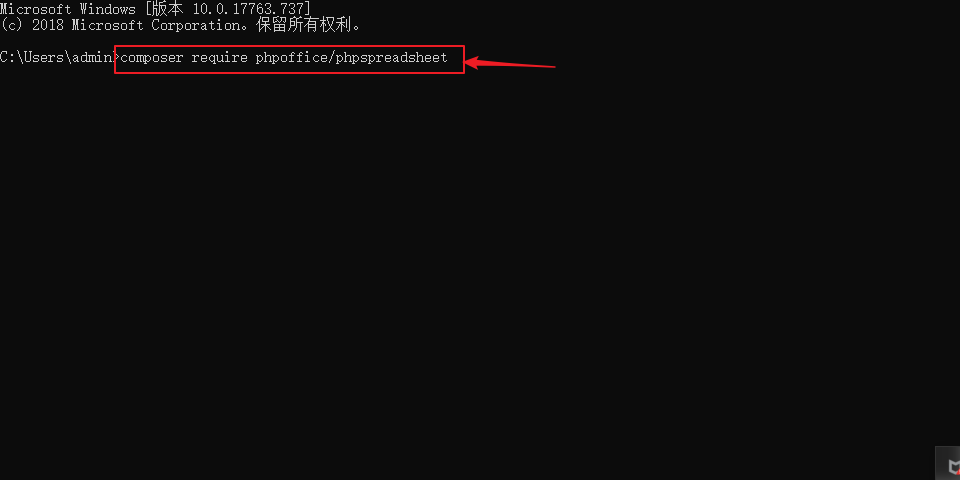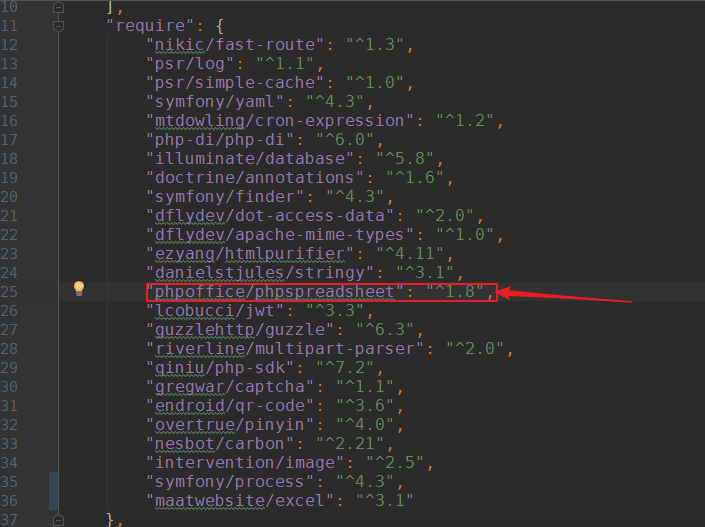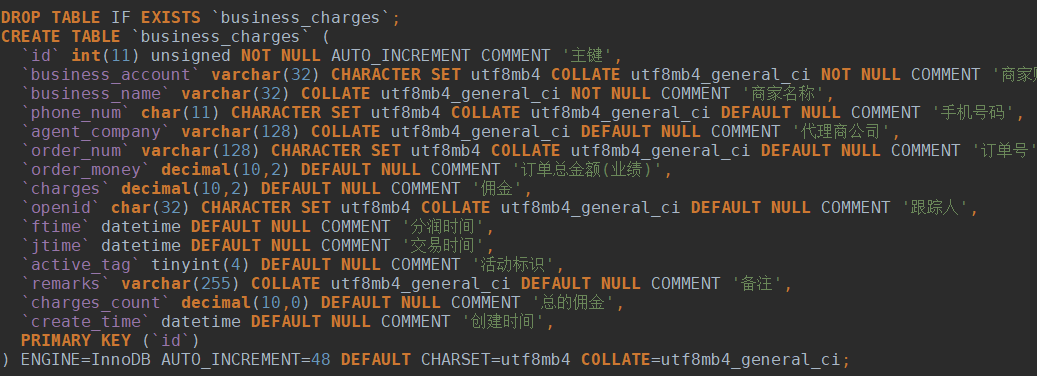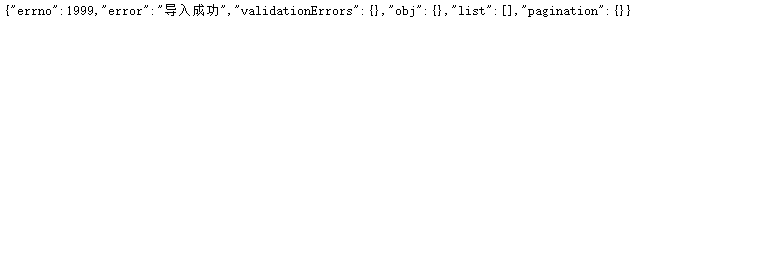• 如何Excel导入成绩到 Moodle 中 ossez.com
• 有时写程序时后台要求把大量数据导入数据库中，比如考试成绩、电话簿等一般都是存放在excel中的数据，这时我们可把excel导出成csv文件，然后通过以下程序即可批量导入数据到数据库中 上传cvs并导入到数据库中，测试...
有时写程序时后台要求把大量数据导入数据库中，比如考试成绩、电话簿等一般都是存放在excel中的数据，这时我们可把excel导出成csv文件，然后通过以下程序即可批量导入数据到数据库中
上传cvs并导入到数据库中，测试成功（部分代码不规范，如PHP_SELF那里要改写成$_SERVER["PHP_SELF"] ） PHP代码 <?php$fname = $_FILES['MyFile']['name'];$do = copy($_FILES['MyFile']['tmp_name'],$fname);
if ($do) { echo"导入数据成功<br>"; } else { echo ""; } ?> <form ENCTYPE="multipart/form-data" ACTION="<?php echo"".$PHP_SELF.""; ?>" METHOD="POST">
<p>导入CVS数据 <input NAME="MyFile" TYPE="file"> <input VALUE="提交" TYPE="submit">
</p>
</form>
<?
error_reporting(0);
//导入CSV格式的文件
$connect=mysql_connect("localhost","a0530093319","123456") or die("could not connect to database"); mysql_select_db("a0530093319",$connect) or die (mysql_error());
$fname =$_FILES['MyFile']['name'];
$handle=fopen("$fname","r");
while($data=fgetcsv($handle,10000,","))
{
$q="insert into test (code,name,date) values ('$data','$data','$data')";
mysql_query($q) or die (mysql_error()); } fclose($handle);
?>
用php将数据库导出成excel，测试完全成功

PHP代码
<?php
$DB_Server = "localhost";$DB_Username = "root";
$DB_Password = "";$DB_DBName = "ishop";
$DB_TBLName = "oi_mall_payment";$savename = date("YmjHis");
$Connect = @mysql_connect($DB_Server, $DB_Username,$DB_Password) or die("Couldn't connect.");
mysql_query("Set Names 'gbk'");
$file_type = "vnd.ms-excel";$file_ending = "xls";
header("Content-Type: application/$file_type;charset=big5"); header("Content-Disposition: attachment; filename=".$savename.".$file_ending"); //header("Pragma: no-cache");$now_date = date("Y-m-j H:i:s");
$title = "数据库名:$DB_DBName,数据表:$DB_TBLName,备份日期:$now_date";

$sql = "Select * from$DB_TBLName";
$ALT_Db = @mysql_select_db($DB_DBName, $Connect) or die("Couldn't select database");$result = @mysql_query($sql,$Connect) or die(mysql_error());

echo("$title\n");$sep = "\t";
for ($i = 0;$i < mysql_num_fields($result);$i++) {
echo mysql_field_name($result,$i) . "\t";
}
print("\n");
$i = 0; while($row = mysql_fetch_row($result)) {$schema_insert = "";
for($j=0;$j<mysql_num_fields($result);$j++) {
if(!isset($row[$j]))
$schema_insert .= "NULL".$sep;
elseif ($row[$j] != "")
$schema_insert .= "$row[$j]".$sep;
else
$schema_insert .= "".$sep;
}
$schema_insert = str_replace($sep."$", "",$schema_insert);
$schema_insert .= "\t"; print(trim($schema_insert));
print "\n";
$i++; } return (true); ?>  展开全文php mysql • 2019年09月18日 17:31:48 黎建湛 原文链接 ： ...sub=C7C62B8099F9419CBE711F447181F5A3 项目需求：需要将一个Excel表格数据如客户信息、学生成绩导入到系统数据库中，然后在系统中进行进一步操作，如... 2019年09月18日 17:31:48 黎建湛 原文链接 ： 链接：http://note.youdao.com/noteshare?id=b0287ac7f2e612a6ae4bc6f7be5f416a&sub=C7C62B8099F9419CBE711F447181F5A3 项目需求：需要将一个Excel表格数据如客户信息、学生成绩表导入到系统数据库中，然后在系统中进行进一步操作，如给导入的客户群发短信，统计学生成绩排名。PHP导入Excel避免了人工录入信息的麻烦和出错，提高效率。 环境支持：php7.0版本以上,Mysql5.5以上，Nginx网站服务器 开发须知：① 数据量过大，导致服务器出现异常，需要缓解服务器处理压力 ② 读取与写入的数据条数需要确定下来 ③ 数据表中字段标识，重复的数据插入返回错误码和信息 ④ 数据库使用InnoDB引擎，开启处理事务 开发准备： ① composer下载并安装 ② 开发框架（Thinkphp\Laravel） ③ 插件 PhpSpreadsheet ④ 需要导入的Excel表 步骤： 首先，安装composer包管理工具,官网地址：https://www.phpcomposer.com/利用composer包安装PHP框架，这里不详细说了 下载 phpspreadsheet 输入命令 composer require phpoffice/phpspreadsheet在框架内部生成composer.json文件，告诉composer您的项目所依赖的包，稍微注意一下你项目的插件位置开始数据库表的创建工作，首先我们需要准备一张MySQL表，表名business_charges，表结构如下：然后将Excel文件放置程序应用目录下。当然，实际应用中，我们一般通过web上传到服务器指定目录下，然后再进行导入数据库操作。所以我取得是上传到服务器上得文件目录名称，当然你们可以使用$_FILE超全局变量来对xlsx文档得获取。
准备工作做好后，我们来开始导入。使用PhpSpreadsheet将读取的Excel表格中的有用信息批量地插入到MySQL表。创建一个控制器	ExcelController.php。
PHP源码：

在这里插入代码片
<?php
namespace app\controller\admin;

use app\annotation\GetMapping;
use app\annotation\JwtAuth;
use app\annotation\PostMapping;
use app\annotation\RequestParam;
use app\controller\BaseController;
use app\exception\ApiException;
use app\util\ValidationUtils;
use Illuminate\Database\Capsule\Manager as Db;
use PhpOffice\PhpSpreadsheet\IOFactory;
use PhpOffice\PhpSpreadsheet\Spreadsheet;

class ExcelController extends BaseController {
/**
* 导入excel表
*
* @PostMapping("/admin/imports")
* @JwtAuth("admin")
* @RequestParam(name="xlsxFile",type="JsonObject")
* @param string $xlsxFile */ public function imports($xlsxFile)
{
// 超时提示错误
set_time_limit(600);
ini_set('memory_limit', '2048M');

ValidationUtils::validate(['xlsxFile'=>$xlsxFile],[ 'xlsxFile@StrNotEmpty|>>>:请上传文件' ]); // 处理文件目录$xlsxFile = _ROOT_."/{$xlsxFile}";$reader = IOFactory::createReader('Xlsx');
$reader->setReadDataOnly(TRUE);$spreadsheet = $reader->load($xlsxFile);
$worksheet =$spreadsheet->getActiveSheet();

// 总行数
$hignestRow =$worksheet->getHighestRow();
// 总列数
$highestColumn =$worksheet->getHighestColumn();
$lines =$hignestRow - 2;

if($lines <= 0){ throw new ApiException("Excel表格中没有数据"); } if($hignestRow >= 500){
throw new ApiException("数据量过大，请删除些数据");
}

// 定义数组
$data = []; for($row = 2; $row <=$hignestRow; ++$row){$phoneNumber = $worksheet->getCellByColumnAndRow(3,$row)->getValue();
$Nowscharges =$worksheet->getCellByColumnAndRow(7,$row)->getValue();$list = Db::table('business_charges')->where('phoneNum','=',$phoneNumber)->get();$setList = $list->toArray();$listPhoneNum = $setList->phoneNum;$data[] = [
'businessAccount' => $worksheet->getCellByColumnAndRow(1,$row)->getValue(),
'businessName' => $worksheet->getCellByColumnAndRow(2,$row)->getValue(),
'phoneNum' => $phoneNumber, 'agentCompany' =>$worksheet->getCellByColumnAndRow(4,$row)->getValue(), 'orderNum' =>$worksheet->getCellByColumnAndRow(5,$row)->getValue(), 'orderMoney' =>$worksheet->getCellByColumnAndRow(6,$row)->getValue(), 'charges' =>$Nowscharges,
'human' => $worksheet->getCellByColumnAndRow(8,$row)->getValue(),
'ftime' => $worksheet->getCellByColumnAndRow(9,$row)->getValue(),
'jtime' => $worksheet->getCellByColumnAndRow(10,$row)->getValue(),
'activeTag' => $worksheet->getCellByColumnAndRow(11,$row)->getValue(),
'remarks' => $worksheet->getCellByColumnAndRow(12,$row)->getValue(),
'createTime' => date('Y-m-d H:i:s')
];
}

// 开启事务
Db::beginTransaction();

$res = Db::table('business_charges')->insert($data);

if($res){ // 提交事务 Db::commit(); throw new ApiException("导入成功"); }else{ // 回滚事务 Db::rollback(); throw new ApiException("导入失败"); } // 删除临时文件 unlink($xlsxFile);

}


$worksheet->getCellByColumnAndRow($col, $row)->getValue()可以获取表格中任意单元格数据内容，$col表示单元格所在的列，以数字表示，A列表示第一列，\$row表示所在的行。最后使用sql语句将其导入到数据库中。
导入成功后，返回提示成功信息展开全文PHP
• 鲁迅先生说过：伟大的成绩和辛勤劳动是成正比例的，有一分劳动就有一分收获，日积月累，从少到多，奇迹就可以创造出来因此，面对Excel图片如何批量导入我们应该有努力探索的精神。你缺少的不是金钱，而是能力，经验...

在工作学习中，我们经常会遇到Excel图片如何批量导入这样的问题。鲁迅先生说过：伟大的成绩和辛勤劳动是成正比例的，有一分劳动就有一分收获，日积月累，从少到多，奇迹就可以创造出来因此，面对Excel图片如何批量导入我们应该有努力探索的精神。你缺少的不是金钱，而是能力，经验和机会。对于这个问题也是一样的。读书忌死读，死读钻牛角，对于Excel图片如何批量导入我们一定要从不同角度去理解，只有这样才能找到解决办法。高尔基告诉我们：一个人追求的目标越高，他的才力就发展得越快，对社会就越有益，解决这个问题会让我们发展的更快。

1、首先我们全选表格，调整表格的列宽行高。
2、然后点击【插入】-【图片】，将导入的图片全部选择插入进来。
3、接着在上方的图片设置栏中设置图片的大小宽度。
4、然后在最后一个单元中首先插入图片。并再次选中所有图片，在【排列】栏中选择【对齐】-【左对齐】，这样所有的图片都会排列整齐。
5、最后一步再次点击【对齐】-【纵向分布】，如此一来，所有的图片都会规整的移动到自己的单元格中，无需你手动去一张一张的去拖动啦。
以上就是LEO老师对于Excel图片如何批量导入的介绍，不知道是否解决了您的问题，欢迎给我留言就Excel图片如何批量导入与我讨论，更多内容请访问我的博客：头条号：https://www.toutiao.com/c/user/500976462869069/


展开全文• 如何将txt文本导入excel实例 这里我们用python中的random.randint方法在txt文本当中随机生成100份成绩 这里我的项目文件名为to_score.py import random #随机写入100个学生的数学,计算机分数到txt文本当中 with ...
如何将txt文本导入excel实例

这里我们用python中的random.randint方法在txt文本当中随机生成100份成绩

这里我的项目文件名为to_score.py
import random

#随机写入100个学生的数学,计算机分数到txt文本当中
with open("score.txt","w") as fw:
num_s = 1
num_e = 100
for i in range(num_s,num_e+1):
#遍历1-100随机生成100份成绩
math = random.randint(10,100)
computer = random.randint(10,100)
#\n是换行符,若有不会使用format方法的请自行查询!
fw.write("{},{},{}\n".format(i,math,computer))
#若with open as 放在这个位置,则read出来的是null值
#因为read()读取时是从指针位置开始读取的.所以这里一边写入数据一边读取数据,读取出来的只有空值.
with open(r"score.txt","r") as fr :
print(fr.read())#read()读取文档全部内容,查看是否写入



以上操作完成之后,你会发现你的项目当中会多出一个score.txt.
这就是随机生成的100份成绩接下来我们新创建一个sheet.py(名字随意)

导入openpyxl包.
利用相关的方法把txt文本导入excel表格

from openpyxl import Workbook
#导入openpyxl包
wb = Workbook()#创建一个excel
sheet = wb.active#获取当前excel的sheet
datas = None#定义一个空值,接收score.txt的参数

with open("score.txt","r") as fr :#读取上面txt的数据,这里我的txt文本命名为score.txt
datas = fr.readlines()

sheet["A1"] = "学号" #excel中:A列第一行,写入"学号"
sheet["B1"] = "数学"
sheet["C1"] = "计算机"

for i in range(len(datas)):#遍历datas的长度,100行
id_,math_,computer_ = datas[i].split(",")
"""
当i等于1时这里的 id_,math,computer=(to_execl.py里的)i,math,computer
以此类推
"""
row = str(i + 2)
sheet["A" + row] = id_
sheet["B" + row] = math_
sheet["C" + row] = computer_

wb.save("score.xlsx") #保存excel名字随意,后缀要注意



这样我们完成了对txt导入excel的相关操作.展开全文python
• 这几天我一直想把excel里面的数据导入到mysql数据库中，这个excel里面包含了300人的成绩，每个同学有十几门课，课程名和课程数量也不相同，一共有上千行的数据，如果手撸的话可能要发疯，于是在网上找帖子终于学会了...
• PhpSpreadsheet如何读取excel文件 一、总结 一句话总结：万能的百度，直接搜代码就好，绝对有，...日常开发中，我们经常遇到这样的场景，需要将一个Excel表格数据如客户信息、学生成绩导入到系统数据库中，然后...
• 今天被一个小问题难住了，本人用自己开发的成绩分析软件统计学校成绩，数据由excel导入，给我的数据全部是文本型，其实也不难，主要是我的软件是早期开发的，没有考虑这个问题，结果这个问题被美女老师解决了，现在...
• 如何利用access 统计成绩： 各班 各分数段 统计（每50人一段） 各科各分数段统计 各分数段成绩范围。 sorce、sort为基本表格(score可由查询sort001导出为excel表格，再重新导入access中)，score表格有班级，座号，...
• 如何利用access 统计成绩：得出总分 平均分 班排名 级排名等等。 sorce为基本表格，有班级，座号，姓名，各科分数等字段。可以利用excel导入。查询sort001为结果。要比较完善的请看第三份，还有高二高三的有分文理科...
• 在做一中考评项目的时候，遇到了这么一个需求，就是把评教后得到的...后来需求变了，要求额外加分项动态添加，也就是说一个老师有几个额外加分项是管理员导入的，这样成绩表就不能用了，因为额外加分项是不固定的。所
• 糸统要求：使用ASP.NET2.0+SQL2000开发，糸统要求可以方便使用EXCEL进行导入.导出,因为是为单个学院开发的， 分类从专业->班级->学生，可供管理员管理学生成绩和基本的个人信息，学生也可以通过糸统查询成绩...
• 16如何避免Excel中的错误信息 17. 不用编程--Excel公式也能计算个人所得税 18. 用EXCEL轻松处理学生成绩 19. 用EXCEL轻松准备考前工作 20. Excel的图表功能 21. 批量修改数据 22. 将Excel数据导入Access 23. ...
• 　我们需要把Excel中的一些资料更新到数据库表中，比如学生的考试系统，在数据中已经有了考生的ID，这里有一份考生ID和考生成绩Excel表，我们如何把考生成绩更新到数据库表中呢？   方案： 我们最常使用...
• 下面我们将分情况讨论如何利用集算器将Excel数据进行结构化。文中用到的函数请参看集算器文档...　集算器处理这种文件也非常简单：A1 打开“学生成绩表.xlsx”文件并导入成序表，选项@t表示文件第一行是列标题 A2 ...
• 如何Excel自动扩展格式 • 如何Excel自动套用格式 • 自定义工具栏 • 非连续区域集中打印 • 设置打印标题 第2篇 使用公式和函数 • 使用简单公式计算产品销售利润 • 使用相对和绝对引用计算产品销售额与...
• 全书分为6篇25章，介绍了Excel数据处理与分析方面的应用技巧，内容涉及Excel工作环境和基本操作、数据的输入和导入、数据整理和编辑、数据查询、分类汇总和合并计算等方面的使用技巧，以及数据透视表、模拟运算表、...
• 任务8： EXCEL基本操作之导入外部数据 任务9： EXCEL基本操作之设置单元格格式、条件格式 任务10： EXCEL基本操作之保护工作表、高级筛选 任务11： 数据验证 任务12： 合并计算之模拟运算 任务13： 合并计算之去重 ...数据分析师
• 以前都是在Excel中做好数据,诸如字段名,各科及总分的名次(还要用rank函数),然后再导入到VFP中进行处理,明显的效率太低,主要是来回的转化实在是烦人.现在可以在Excel中进行处理了. 先看一下原文件是如何布局的,如下...
• 5、学籍导出与会考成绩Excel数据批量导入 6、可以快速批量分班及班级信息维护 7、设计灵活人性，各字段项目完全自定义设置，参与统计的年龄段、中考分数段自定义。 8、回收站功能不怕误删，记录审核功能让记录...
• 1.基础是学生资料管理系统，可以独立出来，甚至改造成学生成绩管理等其他系统 2.表格全部用百分比，自动适应800*600、1024*768、1600*1200等分辨率 3.学生网页外观由student.css控制，老师网页外观由teacher.css...
• 21.7.1 导入Excel相关类 21.7.2 在程序中操作Excel 21.7.3 导入Word相关类 21.7.4 在程序中操作Word 21.8 系统测试 21.9 程序设计清单 21.10 技术、经验总结 21.10.1 技术总结 21.10.2 经验总结 21.11 系统的编译与...
• 21.7.1 导入Excel相关类 21.7.2 在程序中操作Excel 21.7.3 导入Word相关类 21.7.4 在程序中操作Word 21.8 系统测试 21.9 程序设计清单 21.10 技术、经验总结 21.10.1 技术总结 21.10.2 经验总结 21.11 系统的编译与...